# Knowledge Hypergraphs: Prediction Beyond Binary Relations

## Basic Idea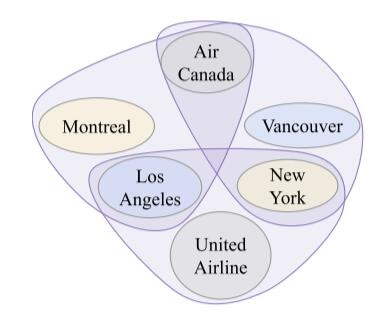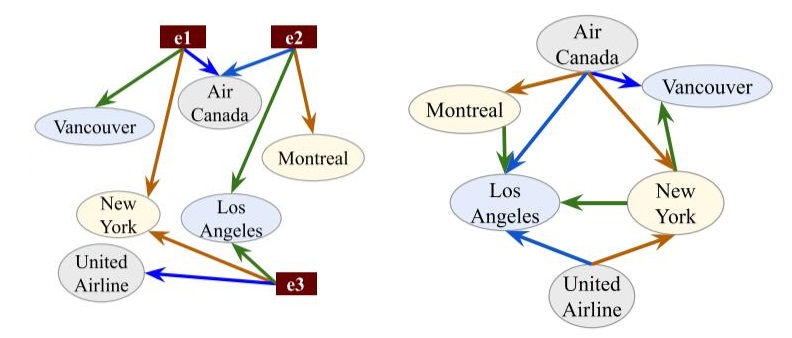## Hypergraph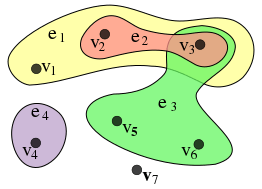\begin{aligned} X&=\{v_1, v_2, v_3, v_4, v_5, v_6, v_7\} \\\ \\ E&=\{e_1, e_2, e_3, e_4\}\\ &=\{\{v_1, v_2, v_3\}, \{v_2, v_3\}, \{v_3, v_5, v_6\}, \{v_4\}\} \end{aligned}

## HypE

$$\odot\left(\mathbf{v}_{\mathbf{1}}, \mathbf{v}_{\mathbf{2}}, \ldots, \mathbf{v}_{\mathbf{k}}\right)=\sum_{i=1}^{\ell} \mathbf{v}_{\mathbf{1}}{ }^{(i)} \mathbf{v}_{\mathbf{2}}{ }^{(i)} \ldots \mathbf{v}_{\mathbf{k}}^{(i)}$$

$\mathbf{v}_j^{(i)}$ 为$\mathbf{v}_j$ 的第$i$ 个元素.

### HSimplE

$$\phi\left(r\left(e_{1}, e_{2}\right)\right)=\odot\left(\mathbf{r}^{(\mathbf{1})}, \mathbf{e}_{\mathbf{1}}^{(\mathbf{1})}, \mathbf{e}_{\mathbf{2}}^{(\mathbf{2})}\right)+\odot\left(\mathbf{r}^{(\mathbf{2})}, \mathbf{e}_{\mathbf{2}}^{(\mathbf{1})}, \mathbf{e}_{\mathbf{1}}^{(\mathbf{2})}\right)$$

\begin{aligned} \phi\left(r\left(e_{i}, e_{j}, \ldots, e_{k}\right)\right) &=\odot\left(\mathbf{r}, \mathbf{e}_{\mathbf{i}}, \operatorname{shift}\left(\mathbf{e}_{\mathbf{j}}, \operatorname{len}\left(\mathbf{e}_{\mathbf{j}}\right)\cdot\frac{1} {\alpha}\right), \ldots,\right. \left.\left.\operatorname{shift}\left(\mathbf{e}_{\mathbf{k}}, \operatorname{len}\left(\mathbf{e}_{\mathbf{k}}\right) \cdot\frac{(\alpha-1)} { \alpha}\right)\right)\right) \end{aligned}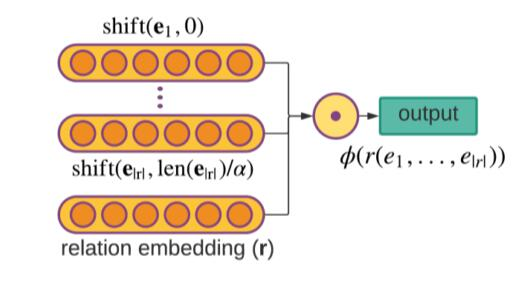### HypE

HSimplE的平移操作有点太简单了, 把HSimplE的Shift操作换成了卷积就是HypE.

$$f(\mathbf{e}, i)=\operatorname{concat}\left(\mathbf{e} \ast \omega_{\mathrm{i} 1}, \ldots, \mathbf{e} \ast \omega_{\mathrm{in}}\right) \mathrm{P}$$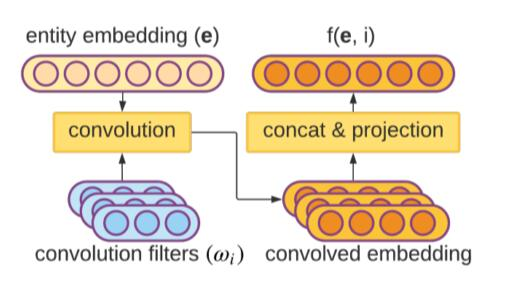$$\phi\left(r\left(e_{1}, \ldots, e_{|r|}\right)\right)=\odot\left(\mathbf{r}, f\left(\mathbf{e}_{\mathbf{1}}, 1\right), \ldots, f\left(\mathbf{e}_{|\mathbf{r}|},|r|\right)\right)$$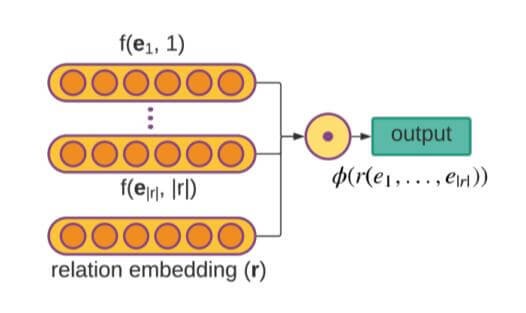1. 由于实体Embedding的位置信息是由卷积核额外添加进去的, 而非包含于实体Embedding本身, 所以更利于实体Embedding变得与位置无关.
2. 位置和实体的分离使得HypE能作用于任意数量实体的Knowledge base, 这额外给予了HypE更多的鲁棒性, 即使遇到从未见过的实体, 也能有点用处.

### Objective Function and Training

HSimplE和HypE都使用小批量梯度下降训练.

$$\mathcal{L}(\mathbf{r}, \mathbf{e})=\sum_{x^{\prime} \in \tau_{\text {train }}^{\prime}}-\log \left(\frac{e^{\phi\left(x^{\prime}\right)}}{e^{\phi\left(x^{\prime}\right)}+\sum_{x \in T_{n e g}\left(x^{\prime}\right)} e^{\phi(x)}}\right)$$

$x$ 为所有训练集, 验证集, 测试集元组$\tau^\prime$ 的某个元组, $\tau ^ \prime_\text{train}$ 为训练集, $T_{neg}(x^\prime)$​ 为生成的负例.

## Experiments

### Dataset

JF - 17K是前人提出的数据集, 但没有验证集, 作者随机选了20%作为验证集.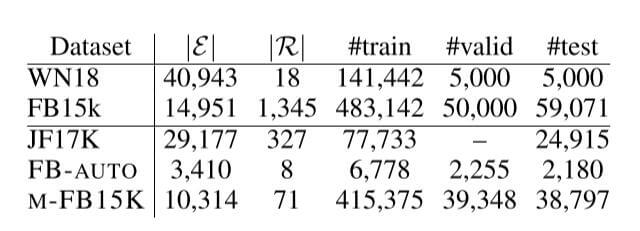### Baseline

• 对于处理二元关系的方法, 作者将其扩展到更多实体的情况, 如r - SimplE, m - DistMult, m - CP.
• 也有一些不用扩展的方法, 能直接处理更多实体, 如m - TransH.

### Knowledge HyperGraph Completion Results

HypE在这几个超图补全数据集上结果如下: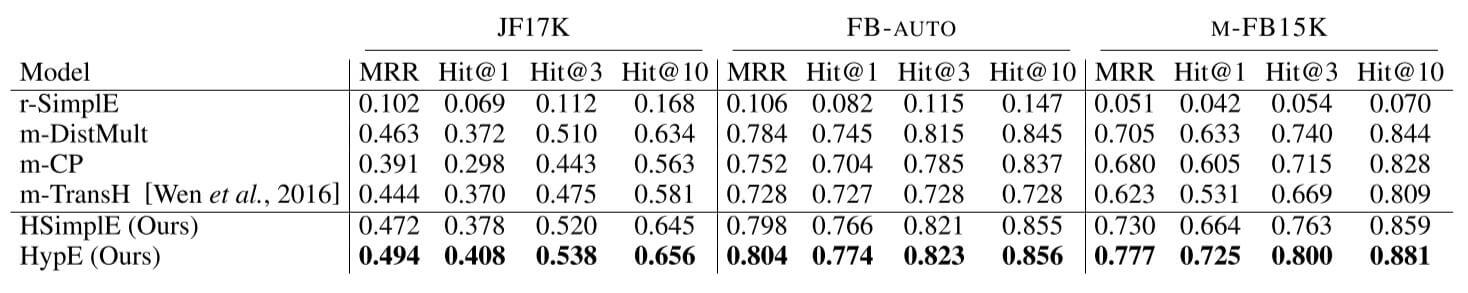HypE相较于其他方法有显著提升.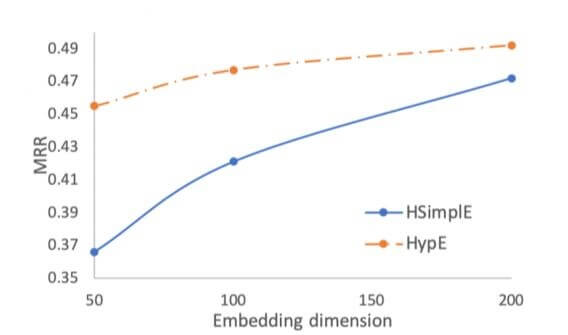HypE在200维度以下时, 是完全优于Baseline HSimplE的.

HypE使用了位置特化的卷积核, 应该能处理更多实体出现在不同位置上的情况, 作者创建一个缺失位置的测试集, 其中的每个元组至少有一个实体在某个位置没有出现过, 这种情况更具挑战性. 与其他方法对比结果如下: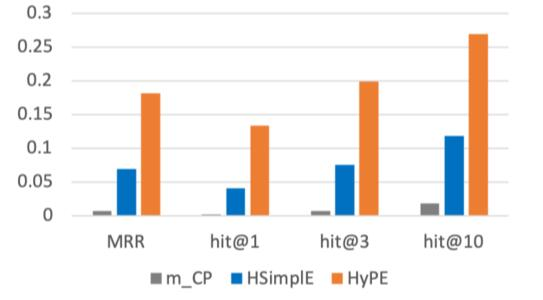### Knowledge Graph Completion Results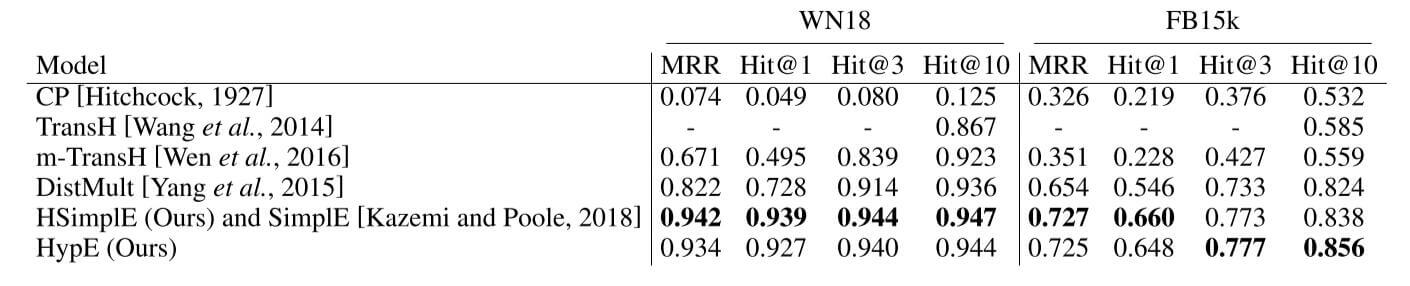HSimplE取得了相当好的表现, HypE也与其相似.

### Ablation Study on Different Arities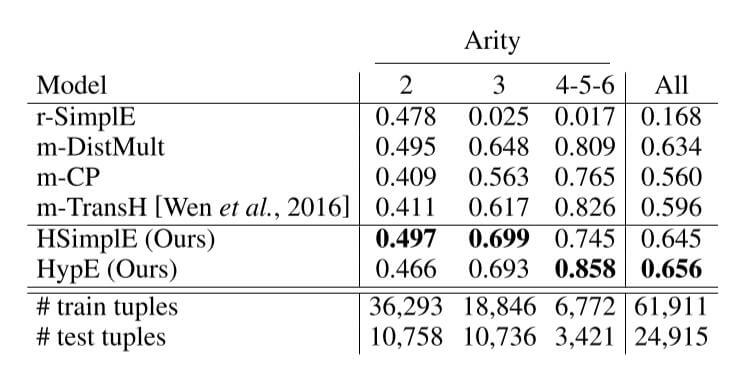## Summary

上一篇A Unified MRC Framework for Named Entity Recognition

2021-09-30Pytorch实现: VAE

2021-07-10
目录